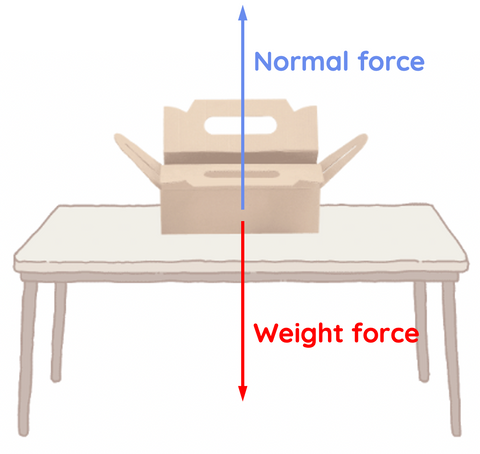# Equilibrium in Physics

This topic is part of the HSC Physics course under the section Forces.

### HSC Physics Syllabus

• explore the concept of net force and equilibrium in one-dimensional and simple two-dimensional contexts using: (ACSPH050)

– vector addition by resolution into components

### What is Equilibrium in Physics?

In HSC Physics, the concept of equilibrium is an essential building block for understanding various physical phenomena. Grasping this concept helps students analyse situations involving forces and their effects on objects. In this article, we will delve into the concept of equilibrium and its relation to net force. By the end of this article, you will have a clear understanding of the role equilibrium plays in physics and how to apply it to various problems.

### What is Equilibrium in Physics?

Equilibrium is a state where an object experiences no change in its motion. It can be categorised into two types: static and dynamic.

Static equilibrium occurs when an object is at rest and not moving, while dynamic equilibrium occurs when an object is in constant motion with no change in velocity. Both types of equilibrium involve balancing forces, which brings us to the concept of net force.

### Net Force and Its Role in Equilibrium

Net force is the vector sum of all the individual forces acting on an object. When the net force is zero, an object is said to be in equilibrium. In other words, the forces acting on the object are balanced, leading to no change in its motion. The concept of net force is discussed in greater detail here.

For equilibrium to exist, net force must equal zero.

### Static Equilibrium

In static equilibrium, an object is at rest and not moving. For this to occur, two conditions must be met:

1. The sum of all the forces acting on the object in the horizontal direction must be zero.
2. The sum of all the forces acting on the object in the vertical direction must also be zero.

This ensures that the net force in both directions is zero, and there is no acceleration or change in motion.Example: Consider a book resting on a table. The forces acting on the book are the gravitational force (weight) acting downward and the normal force exerted by the table acting upward. These forces are equal in magnitude but opposite in direction, resulting in a net force of zero. Thus, the book is in static equilibrium.

Mathematically, the forces in this static equilibrium can be expressed as:

$$F_{net} = F_{normal} - mg$$

### Dynamic Equilibrium

Dynamic equilibrium occurs when an object is in constant motion with no change in velocity. This means that the object's acceleration is zero, and the net force acting on the object is also zero. In dynamic equilibrium, the forces acting on the object are balanced, just like in static equilibrium, but the object is in motion.Example: Imagine a car moving at a constant speed on a straight, horizontal road. The forward force from the engine balances the friction and air resistance acting in the opposite direction. In this case, the net force is zero, and the car is in dynamic equilibrium.

Mathematically, the net force in the y-direction can be expressed as:

$$Fy_{net} = F_{normal} - mg$$

$$0 = F_{normal} - mg$$

$$F_{normal} = mg$$

Similarly, the net force in the x-direction can be expressed as:

$$Fx_{net} = F_{driving} - F_{friction} - F_{air}$$

$$0 = F_{driving} - F_{friction} - F_{air}$$

### Applying Equilibrium Concepts in Physics

Understanding equilibrium and net force is crucial for solving various physics problems. When analysing a situation, always start by identifying the forces acting on the objects involved. Then, determine if the forces are balanced or unbalanced. If the forces are balanced, the object is in equilibrium, and its motion will remain unchanged.